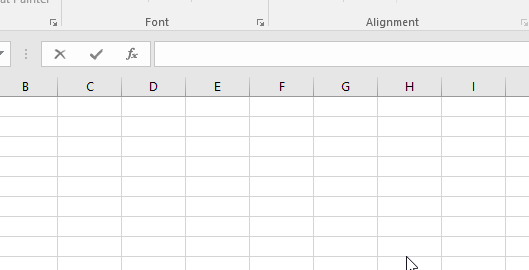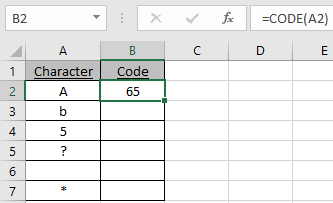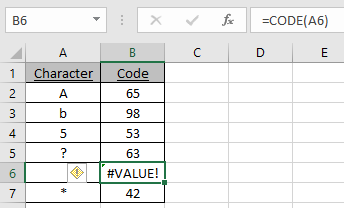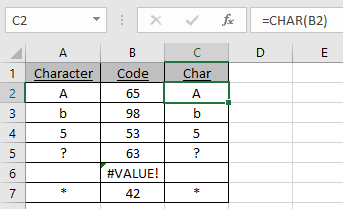# How to use the CODE Function in Excel

In this article, we will learn about how to use the CODE function in Excel.

The CODE function returns the ANSI code (American National Standard Institute's code) of any given character in Excel.
Syntax:

=CODE (“character”)

character : in quotes
Cell reference: not in quotes

Let’s understand this function using it in an example.As you can see in the above snapshot the CODE function takes the character(“A”) in quotes as an argument and returns the numeric code for the character which is 65.

Now we will understand it using cell references in the formula.

Here we have a list of characters we need the code of
Characters using the function.Use the formula:

=CODE(A2)

A2 : character at A2As you can see the code of the character is calculated using the CODE function in Excel
Copy the formula in other cells using shortcut Ctrl + D.The function returns a Value error if empty cell is given as an argument.

You can use the CHAR function to get the character out of a code.As you can see the CODE function returns the ANSI code & CHAR function returns the character from CODE function.

Hope you understood how to use the CODE function and referring cell in Excel. Explore more articles on Excel info functions here. Please feel free to state your query or feedback for the above article.

Related Articles:

How to use the CHAR Function in Excel

How to use the UNICHAR Function in Excel

How to use the UNICODE Function in Excel

Popular Articles:

How to use the VLOOKUP Function in Excel

How to use the COUNTIF function in Excel 2016

How to Use SUMIF Function in Excel

Terms and Conditions of use

The applications/code on this site are distributed as is and without warranties or liability. In no event shall the owner of the copyrights, or the authors of the applications/code be liable for any loss of profit, any problems or any damage resulting from the use or evaluation of the applications/code.